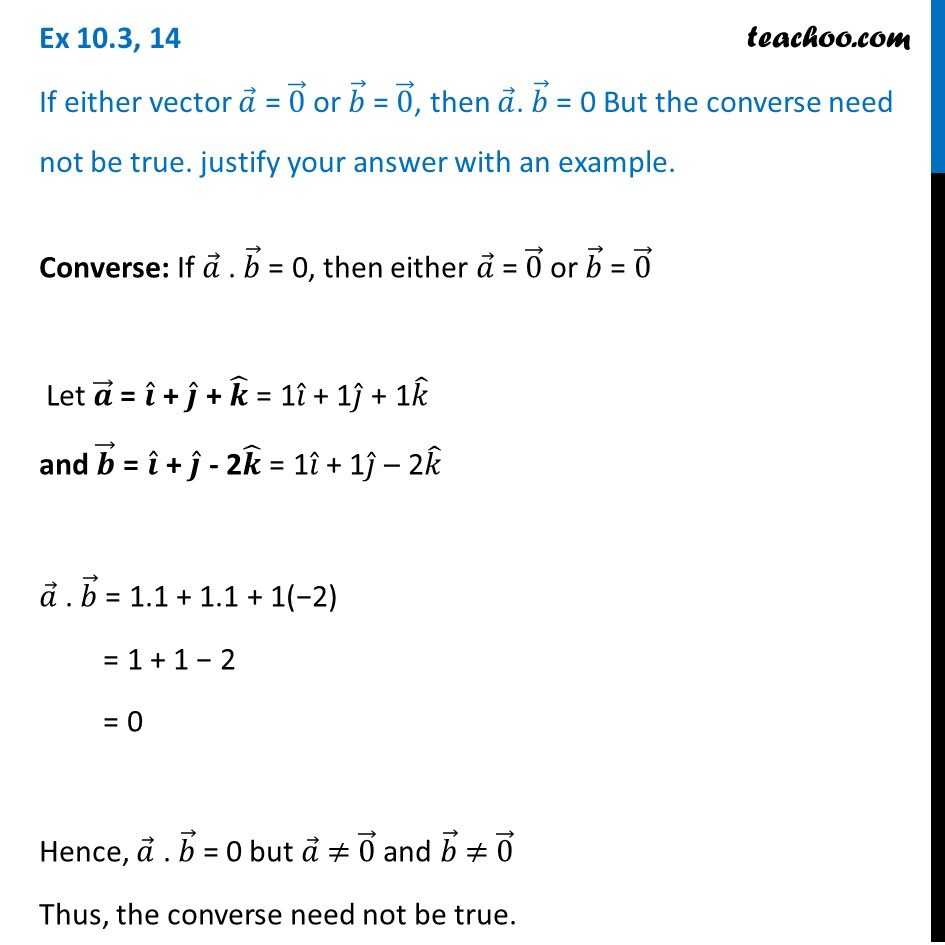Ex 10.3

Chapter 10 Class 12 Vector Algebra
Serial order wiseLearn in your speed, with individual attention - Teachoo Maths 1-on-1 Class

### Transcript

Ex 10.3, 14 If either vector 𝑎 ⃗ = 0 ⃗ or 𝑏 ⃗ = 0 ⃗, then 𝑎 ⃗. 𝑏 ⃗ = 0 But the converse need not be true. justify your answer with an example. Converse: If 𝑎 ⃗ . 𝑏 ⃗ = 0, then either 𝑎 ⃗ = 0 ⃗ or 𝑏 ⃗ = 0 ⃗ Let 𝒂 ⃗ = 𝒊 ̂ + 𝒋 ̂ + 𝒌 ̂ = 1𝑖 ̂ + 1𝑗 ̂ + 1𝑘 ̂ and 𝒃 ⃗ = 𝒊 ̂ + 𝒋 ̂ - 2𝒌 ̂ = 1𝑖 ̂ + 1𝑗 ̂ – 2𝑘 ̂ 𝑎 ⃗ . 𝑏 ⃗ = 1.1 + 1.1 + 1(−2) = 1 + 1 − 2 = 0 Hence, 𝑎 ⃗ . 𝑏 ⃗ = 0 but 𝑎 ⃗ ≠ 0 ⃗ and 𝑏 ⃗ ≠ 0 ⃗ Thus, the converse need not be true.